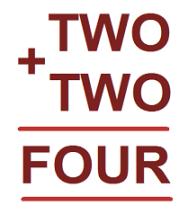#### You may also like### Double Digit### DOTS Division

Take any pair of two digit numbers x=ab and y=cd where, without loss of generality, ab > cd . Form two 4 digit numbers r=abcd and s=cdab and calculate: {r^2 - s^2} /{x^2 - y^2}.### Novemberish

a) A four digit number (in base 10) aabb is a perfect square. Discuss ways of systematically finding this number. (b) Prove that 11^{10}-1 is divisible by 100.

# Two and Two

##### Age 11 to 16Challenge Level

Each of the different letters below stands for a different number.How many solutions can you find to this cryptarithm?
How can you be sure you have found them all?

Can you create other similar cryptarithms?Here are some suggestions to start you off.

ONE + ONE = TWO

ONE + TWO = THREE

ONE + THREE = FOUR

FOUR + FIVE = NINE

Can you make a cryptarithm subtraction?

If you enjoyed this problem you may like to take a look at Cryptarithms